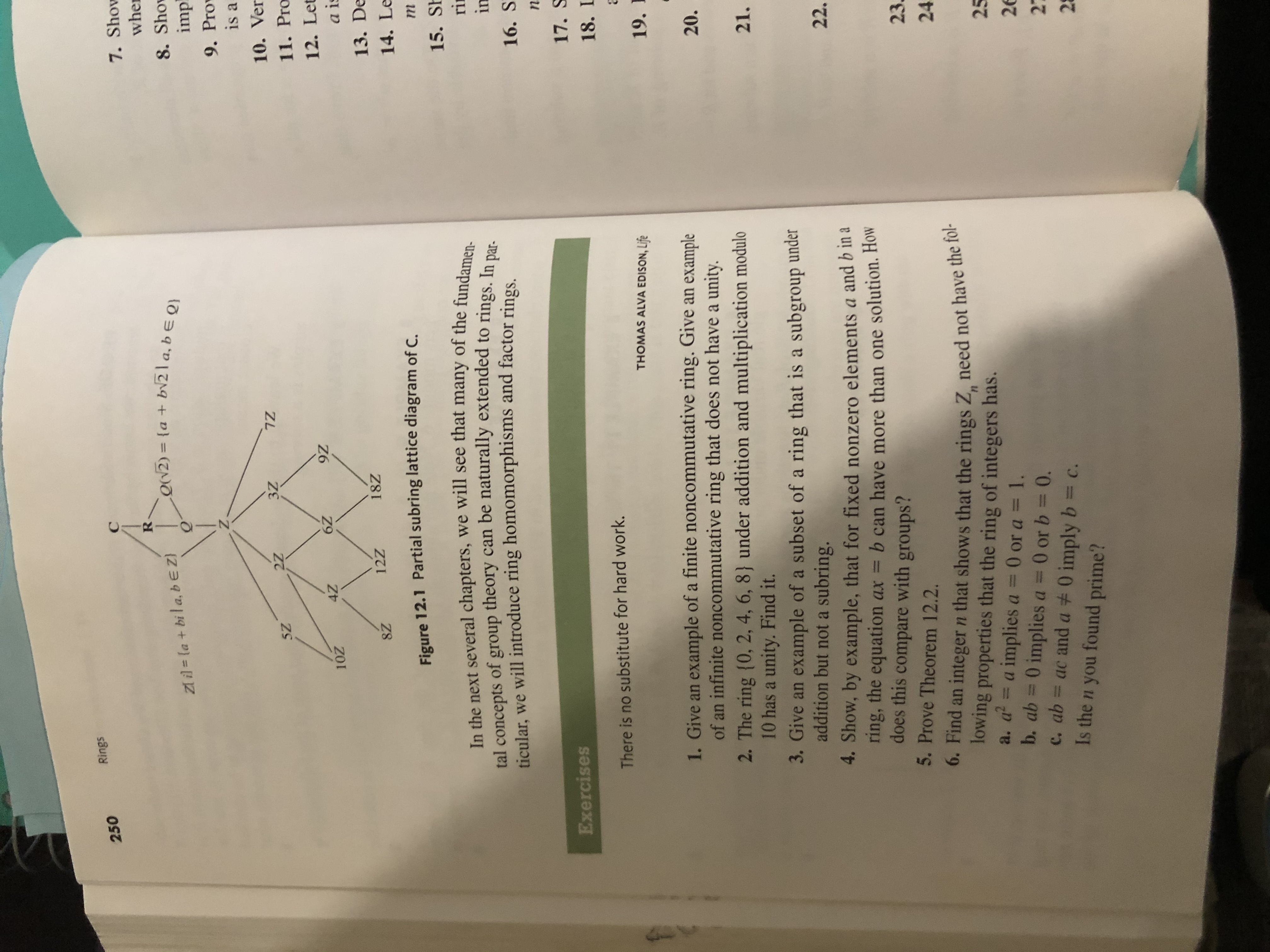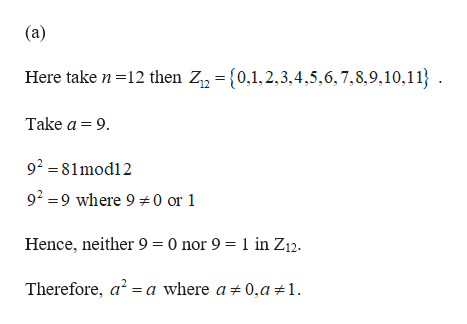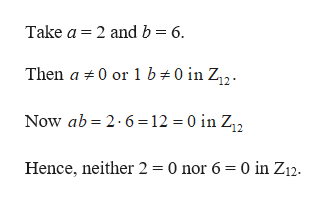# Rings250C7. ShowwheRoN2(a +b12la, b E Qzt il la + bila, bE ZQ8. Shoimp9. ProvZ.is a10. Ver7Z3Z2Z5Z11. Pro12. Let9Z6Z4Z10Za is13. De18Z12Z8Z14. LeFigure 12.1 Partial subring lattice diagram of C.m15. ShIn the next several chapters, we will see that many of the fundamen-riingroup theory can be naturally extended to rings. In par-oftal conceptsticular, we will introduce ring homomorphisms and factor rings16. Sn17. SExercises18. LThere is no substitute for hard work.THOMAS ALVA EDISON, Life19.1. Give an example of a finite noncommutative ring. Give an exampleof an infinite noncommutative ring that does not have a unity2. The ring (0, 2, 4, 6, 8) under addition and multiplication modulo10 has a unity. Find it.3. Give an example of a subset of a ring that is a subgroup underaddition but nota subring.20.21.22.4. Show, by example, that for fixed nonzero elements a and b in aring, the equation axdoes this compare with groups?b can have more than one solution. How235. Prove Theorem 12.2.6. Find an integer n that shows that the rings Z, need not have the fol-lowing properties that the ring of integers has.a. a = a implies a = 0 or a = 1.b. ab 0 implies a = 0 or b = 0.c. ab ac and a 0 imply b c.Is the n you found prime?2526272224

Question
1 views

6help_outlineImage TranscriptioncloseRings 250 C 7. Show whe R oN2 (a +b12la, b E Q zt il la + bila, bE Z Q 8. Sho imp 9. Prov Z. is a 10. Ver 7Z 3Z 2Z 5Z 11. Pro 12. Let 9Z 6Z 4Z 10Z a is 13. De 18Z 12Z 8Z 14. Le Figure 12.1 Partial subring lattice diagram of C. m 15. Sh In the next several chapters, we will see that many of the fundamen- ri in group theory can be naturally extended to rings. In par- of tal concepts ticular, we will introduce ring homomorphisms and factor rings 16. S n 17. S Exercises 18. L There is no substitute for hard work. THOMAS ALVA EDISON, Life 19. 1. Give an example of a finite noncommutative ring. Give an example of an infinite noncommutative ring that does not have a unity 2. The ring (0, 2, 4, 6, 8) under addition and multiplication modulo 10 has a unity. Find it. 3. Give an example of a subset of a ring that is a subgroup under addition but nota subring. 20. 21. 22. 4. Show, by example, that for fixed nonzero elements a and b in a ring, the equation ax does this compare with groups? b can have more than one solution. How 23 5. Prove Theorem 12.2. 6. Find an integer n that shows that the rings Z, need not have the fol- lowing properties that the ring of integers has. a. a = a implies a = 0 or a = 1. b. ab 0 implies a = 0 or b = 0. c. ab ac and a 0 imply b c. Is the n you found prime? 25 26 27 2 224 fullscreen
check_circle

Step 1

It is known that the ring Zn is an integral domain if and only if n is prime.help_outlineImage Transcriptionclose(a) Here take n 12 then Zn = {0,1,2,3.4,5,6,7,8,9,10,11} Take a 9 92 81mod12 92 9 where 9 0 or 1 Hence, neither 9 0 nor 9 = 1 in Z12. Therefore, a2 a where a 0,a 1. fullscreen
Step 2

(b)

Given that ab = 0 implies a = 0 or b =...help_outlineImage TranscriptioncloseTake a 2 and b 6 Then a 0 or 1 b 0 in Z12 Now ab 2 6 12 0 in Z2 Hence, neither 2 = 0 nor 6 = 0 in Z12. fullscreen

### Want to see the full answer?

See Solution

#### Want to see this answer and more?

Solutions are written by subject experts who are available 24/7. Questions are typically answered within 1 hour.*

See Solution
*Response times may vary by subject and question.
Tagged in